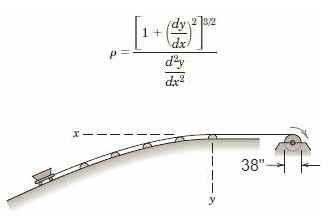# Finding the acceleration in a curvilinear motion (n-t)

Zang

## Homework Statement

The mine skip is being hauled to the surface over the curved track by the cable wound around the 38-in. drum, which turns at the constant clockwise speed of 96 rev/min. The shape of the track is designed so that y = x2/28, where x and y are in feet. Calculate the magnitude of the total acceleration of the skip as it reaches a level of 3.5 ft below the top. Neglect the dimensions of the skip compared with those of the path. Recall that the radius of curvature is given byan = v2

## The Attempt at a Solution

I assumed that the angular speed of the drum is the same as the speed of the mine skip which is constant, so tangential acceleration would be 0.
v = 96 rev/min = 15.92 ft/s.
ρ = 15.33 ft using the given formula.
an = 15.922/15.33 = 16.53 ft/s2.
since at = 0 => a = an = 16.53 ft/s2, but it said my answer is wrong. Is that because at is not 0 and how do I find it?

Orodruin
Staff Emeritus
Homework Helper
Gold Member
If the tangential acceleration was zero the skip would not speed up ...

Zang
If the tangential acceleration was zero the skip would not speed up ...
It didn't say the skip speeds up and doesn't the speed of the skip equal to the angular speed?

Orodruin
Staff Emeritus
Homework Helper
Gold Member
It didn't say the skip speeds up and doesn't the speed of the skip equal to the angular speed?
I missed the drum and cable and thought it was accelerating due to gravity.

Can you show your work leading up to ##\rho = 15.33'##? I do not get the same result.

Zang
I missed the drum and cable and thought it was accelerating due to gravity.

Can you show your work leading up to ##\rho = 15.33'##? I do not get the same result.
y = x2/28
dy/dx = x/14
at x = 3.5 ft, dy/dx = 3.5/14 = .25
d2y/dx2 = 1/14
ρ = ((1+.252)3/2)/(1/14) = (1.0625)3/2 * 14 = 1.095 * 14 = 15.33 ft

Orodruin
Staff Emeritus
•